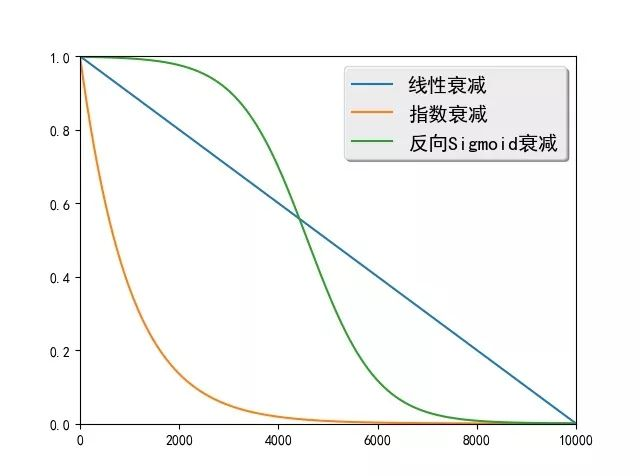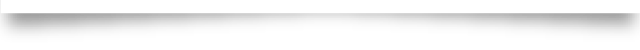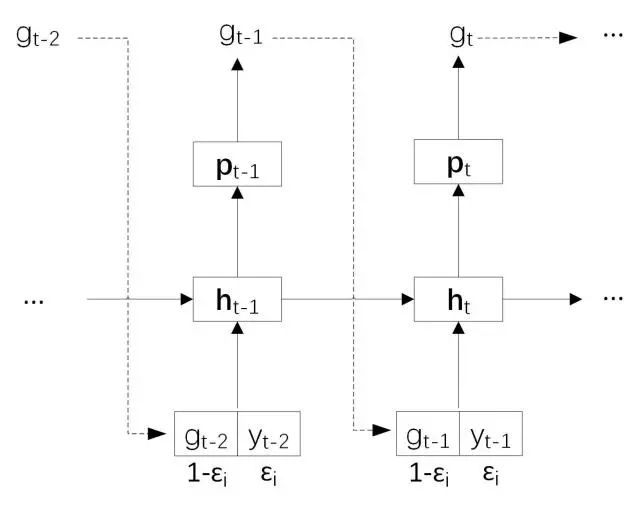# 【序列到序列学习】使用Scheduled Sampling改善翻译质量

2018/03/14 21:17

3.12：【命名实体识别】

3.13：【序列到序列学习】

3.14：【序列到序列学习】

3.15：【序列到序列学习】

3.16：【序列到序列学习】

生成古诗词

【序列到序列学习】

02

|1. 概述

Scheduled Sampling 是一种解决训练和生成时输入数据分布不一致的方法。在训练早期该方法主要使用目标序列中的真实元素作为解码器输入，可以将模型从随机初始化的状态快速引导至一个合理的状态。随着训练的进行，该方法会逐渐更多地使用生成的元素作为解码器输入，以解决数据分布不一致的问题。

|2. 算法简介

Scheduled Sampling主要应用在序列到序列模型的训练阶段，而生成阶段则不需要使用。

• 线性衰减：ϵi=max(ϵ,k−c∗i)，其中ϵ限制ϵi的最小值，k和c控制线性衰减的幅度。

• 指数衰减：ϵi=ki，其中0<k<1，k控制着指数衰减的幅度。

• 反向Sigmoid衰减：ϵi=k/(k+exp(i/k))，其中k>1，k同样控制衰减的幅度。|3. 模型实现

import numpy as np

import math

class RandomScheduleGenerator:

"""

The random sampling rate for scheduled sampling algoithm, which uses devcayed

sampling rate.

"""

...

__init__方法对类进行初始化，其schedule_type参数指定了使用哪种衰减方式，可选的方式有constantlinearexponentialinverse_sigmoidconstant指对所有的mini-batch使用固定的ϵi，linear指线性衰减方式，exponential表示指数衰减方式，inverse_sigmoid表示反向Sigmoid衰减。__init__方法的参数ab表示衰减方法的参数，需要在验证集上调优。self.schedule_computers将衰减方式映射为计算ϵi的函数。最后一行根据schedule_type将选择的衰减函数赋给self.schedule_computer变量。

def __init__(self, schedule_type, a, b):

"""

schduled_type: is the type of the decay. It supports constant, linear,

exponential, and inverse_sigmoid right now.

a: parameter of the decay (MUST BE DOUBLE)

b: parameter of the decay (MUST BE DOUBLE)

"""

self.schedule_type = schedule_type

self.a = a

self.b = b

self.data_processed_ = 0

self.schedule_computers = {

"constant": lambda a, b, d: a,

"linear": lambda a, b, d: max(a, 1 - d / b),

"exponential": lambda a, b, d: pow(a, d / b),

"inverse_sigmoid": lambda a, b, d: b / (b + math.exp(d * a / b)),

}

assert (self.schedule_type in self.schedule_computers)

self.schedule_computer = self.schedule_computers[self.schedule_type]

getScheduleRate根据衰减函数和已经处理的数据量计算ϵi。

def getScheduleRate(self):
"""    Get the schedule sampling rate. Usually not needed to be called by the users    """
return self.schedule_computer(self.a, self.b, self.data_processed_)

processBatch方法根据概率值ϵi进行采样，得到indexesindexes中每个元素取值为0的概率为ϵi，取值为1的概率为1−ϵi。indexes决定了解码器的输入是真实元素还是生成的元素，取值为0表示使用真实元素，取值为1表示使用生成的元素。

def processBatch(self, batch_size):

"""

Get a batch_size of sampled indexes. These indexes can be passed to a

MultiplexLayer to select from the grouth truth and generated samples

from the last time step.

"""

rate = self.getScheduleRate()

numbers = np.random.rand(batch_size)

indexes = (numbers >= rate).astype('int32').tolist()

self.data_processed_ += batch_size

return indexes

Scheduled Sampling需要在序列到序列模型的基础上增加一个输入true_token_flag，以控制解码器输入。

name='true_token_flag',

schedule_type="linear",

decay_a=0.75,

decay_b=1000000):

"""

Creates a data reader for scheduled sampling.

Output from the iterator that created by original reader will be

appended with "true_token_flag" to indicate whether to use true token.

:param schedule_type: the type of sampling rate decay.

:type schedule_type: str

:param decay_a: the decay parameter a.

:type decay_a: float

:param decay_b: the decay parameter b.

:type decay_b: float

:return: the new reader with the field "true_token_flag".

:rtype: callable

"""

schedule_generator = RandomScheduleGenerator(schedule_type, decay_a, decay_b)

for src_ids, trg_ids, trg_ids_next in reader():

yield src_ids, trg_ids, trg_ids_next, \

 + schedule_generator.processBatch(len(trg_ids) - 1)

def gru_decoder_with_attention_train(enc_vec, enc_proj, true_word,

true_token_flag):

"""

The decoder step for training.

:param enc_vec: the encoder vector for attention

:type enc_vec: LayerOutput

:param enc_proj: the encoder projection for attention

:type enc_proj: LayerOutput

:param true_word: the ground-truth target word

:type true_word: LayerOutput

:param true_token_flag: the flag of using the ground-truth target word

:type true_token_flag: LayerOutput

:return: the softmax output layer

:rtype: LayerOutput

"""

name='gru_decoder', size=decoder_size, boot_layer=decoder_boot)

encoded_sequence=enc_vec,

encoded_proj=enc_proj,

decoder_state=decoder_mem)

name='gru_out', size=target_dict_dim)

input=generated_word,

size=word_vector_dim,

input=[true_token_flag, true_word, generated_word_emb])

input=[context, current_word],

size=decoder_size * 3,

bias_attr=False)

name='gru_decoder',

input=decoder_inputs,

output_mem=decoder_mem,

size=decoder_size)

name='gru_out',

input=gru_step,

size=target_dict_dim,

return out

【参考文献】

1. Bengio S, Vinyals O, Jaitly N, et al. Scheduled sampling for sequence prediction with recurrent neural networks//Advances in Neural Information Processing Systems. 2015: 1171-1179.（如欲了解详情，在后台回复当日日期数字，例如“314”！）1.普华永道发布人工智能报告，对人工智能在2018年的发展做出了8项预测。（AI视点）

2.上交大发布知识图谱AceKG，超1亿实体，近100G数据。（新智元）

3.第四范式业界首推免费智能客服服务。（机器之心）end

*原创贴，版权所有，未经许可，禁止转载

*欢迎在留言区分享您的观点

*为了方便大家问题的跟进解决，我们采用Github Issue来采集信息和追踪进度。大家遇到问题请搜索Github Issue，问题未解决请优先在Github Issue上提问，有助于问题的积累和沉淀### 作者的其它热门文章

0
0 收藏

0 评论
0 收藏
0